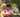### Tape Equilibrium

January 16, 2020

Tape Equilibrium

A non-empty array A consisting of N integers is given. Array A represents numbers on a tape. Any integer P, such that 0 < P < N, splits this tape into two non-empty parts:
A, A, ..., A[P − 1] and A[P], A[P + 1], ..., A[N − 1].
The difference between the two parts is the value of:
|(A + A + ... + A[P − 1]) − (A[P] + A[P + 1] + ... + A[N − 1])|
In other words, it is the absolute difference between the sum of the first part and the sum of the second part.

For example, consider array A such that:
A = 3 A = 1 A = 2 A = 4 A = 3
We can split this tape in four places:
• P = 1, difference = |3 − 10| = 7
• P = 2, difference = |4 − 9| = 5
• P = 3, difference = |6 − 7| = 1
• P = 4, difference = |10 − 3| = 7

Write a function:
function solution(A);
that, given a non-empty array A of N integers, returns the minimal difference that can be achieved. For example, given:
A = 3 A = 1 A = 2 A = 4 A = 3
the function should return 1, as explained above.

Write an efficient algorithm for the following assumptions:
• N is an integer within the range [2..100,000];
• each element of array A is an integer within the range [−1,000..1,000].

Calculate all of the possible differences between the left and right parts.

``````function solution(A) {
// write your code in JavaScript (Node.js 8.9.4)
var smallest = Number.POSITIVE_INFINITY
var sumL=0, sumR=0, temp=0, i

for(i=0; i<A.length; i++) sumR += A[i] // total

for(i=1; i<A.length; i++){ //minimum 2 elements
sumL += A[i-1]
sumR -= A[i-1]
temp = Math.abs(sumL - sumR)
console.log("temp: ", temp)
if(temp < smallest) smallest = temp
}
//console.log("smallest: ", smallest)
return smallest
}

const A0 = [3, 1, 2, 4, 3]
console.log("smallest: ", solution(A0))``````Posted by Tai Lu ( 呂台生 ) who's building useful things.You should follow him on Twitter